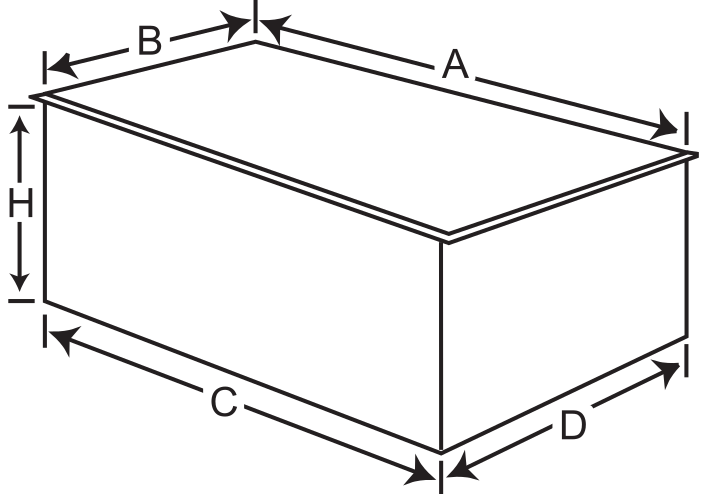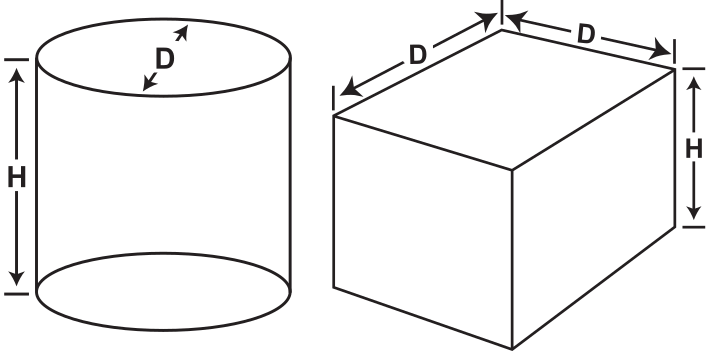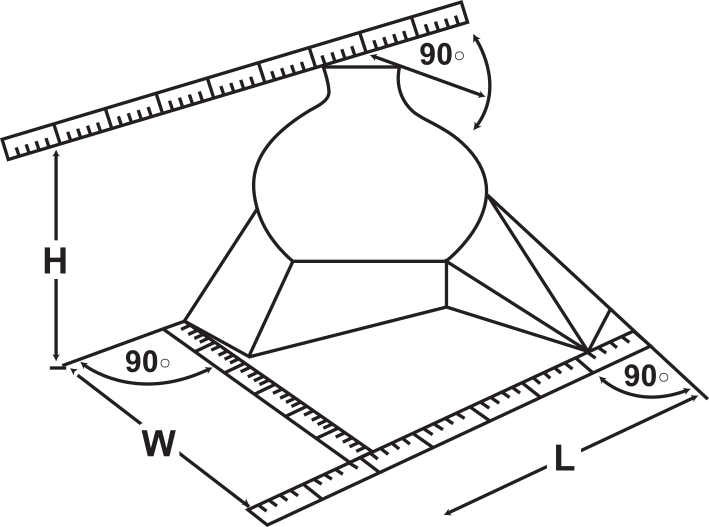## MEASURING OVERLAPPING EDGES

• Measure to the nearest inch (centimeter).
• Use the greatest measurement in each direction.
• For example: Measure A x B x H, not C x D x H## MEASURING CYLINDERS

• Calculate the volume using the diameter as the length and width.
• For example: Measure D x D x H
• The box has the same dimensional weight as the cylinder.## MEASURING IRREGULAR SHAPES

• Place the package in a corner in the position it will be loaded. Measure by holding a straight edge at the right angles to the wall.
• For example: Measure L x W x H## TO CALCULATE VOLUME (EXAMPLES OF CALCULATION)

 A shipment weighs 10 lbs A shipment weighs 10 kgs It measures 14 in by 16 in x 16 in It measures 45 cm by 45 cm x 50 cm (14 x 16 x 16) = 21.59 lbs (45 x 45 x 50) = 16.8 kgs 14 x 16 x 16 = 3584/166 = 21.59 45 x 45 x 50 = 101250/6000 = 16.8

The formula for calculating the dimensional weight for all commodities is 166 cubic inches per pound(6000 cubic centimeters per kilogram or 366 cubic inches per kilogram). Multiply the length by the width by the height to obtain the cubic inches (cm).

• To obtain the dimensional weight in kilograms, divide the cubic inch result by 366.
• To obtain the dimensional weight in pounds, divide the cubic inch result by 166.
• To obtain the dimensional weight in kilograms, divide the cubic centimeter result by 6000.

If the dimensional weight is greater than the actual weight, use it to calculate the shipping charges.